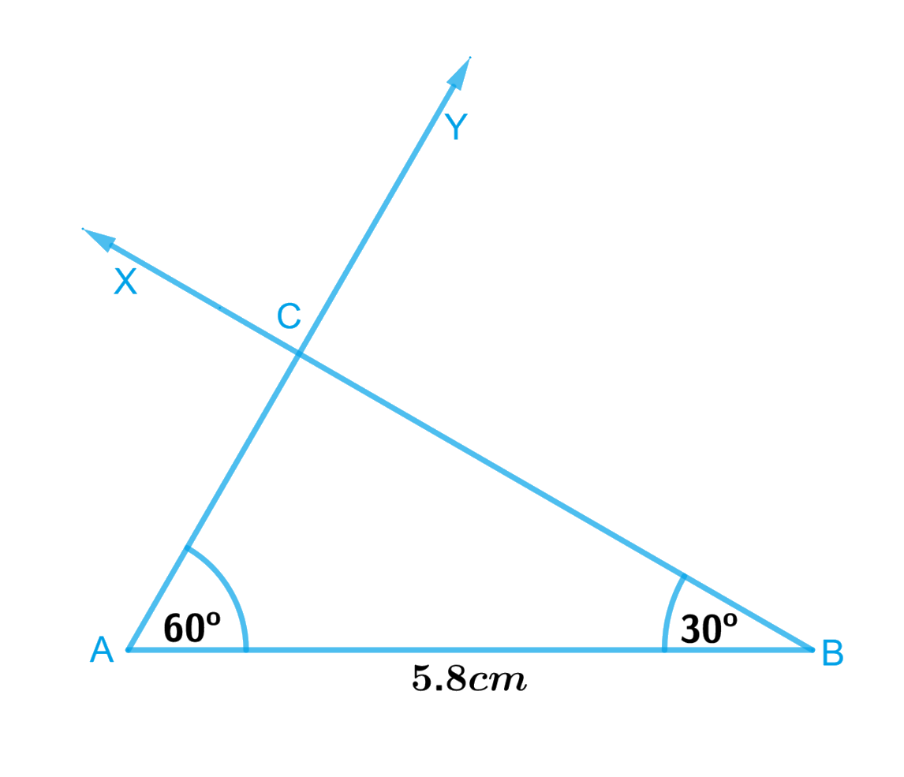# Ex.10.4 Q1 Practical Geometry Solution- NCERT Maths Class 7

Go back to  'Ex.10.4'

## Question

Construct $$ΔABC$$, given $$m∠A = 60^\circ$$, $$m∠B = 30^\circ$$ and $$AB = 5.8\,\rm{ cm}$$.

Video Solution
Practical Geometry
Ex 10.4 | Question 1

## Text Solution

What is known?

Length of side of a $$ΔABC$$, $$AB = 5.8\,\rm{ cm}$$  and  $$∠A = 60^\circ$$, $$∠B = 30^\circ$$.

To construct:

A triangle $$ΔABC$$, given $$∠A = 60^\circ$$, $$∠B = 30^\circ$$ and $$AB = 5.8\,\rm{ cm}$$.

Reasoning:

To Construct a $$ΔABC$$ such that $$∠A = 60^\circ, ∠B = 30^\circ$$ and $$AB = 5.8 \,\rm{cm}$$., follow the steps given below.

Steps:Steps of construction:

1. Draw a line segment $$AB$$ of length $$5.8\,\rm{ cm}.$$
2. At $$A$$, draw ray $$AY$$ making $$60^\circ$$ with $$AB$$.
3. At $$B$$, draw ray $$BX$$ making $$30^\circ$$ with $$AB$$.
4. Rays $$BX$$ and $$AY$$ will intersect at point $$C$$.

Triangle $$ABC$$ is now completed.

Learn from the best math teachers and top your exams

• Live one on one classroom and doubt clearing
• Practice worksheets in and after class for conceptual clarity
• Personalized curriculum to keep up with school